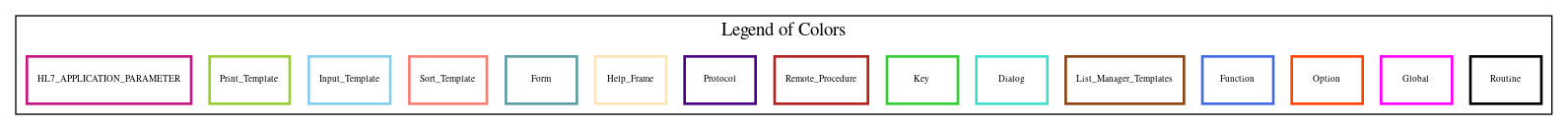Home   Package List   Routine Alphabetical List   Global Alphabetical List   FileMan Files List   FileMan Sub-Files List   Package Component Lists   Package-Namespace Mapping
Routine: PRCHDAM5

# Routine: PRCHDAM5

## Information

PRCHDAM5 ;WISC/DJM-PRINT AMENDMENT,ROUTINE #5 ;6/29/00 12:18

## Source Information

Source file <PRCHDAM5.m>

## Call Graph### Call Graph Total: 1

Package Total Call Graph
IFCAP 1 (DATA,LCNT,LCNT1,LINE)^PRCHDAM4

## Caller Graph

### Legends:#### Package Component Superscript legend

 action A extended action Ea event driver Ed subscriber Su protocol O limited protocol LP run routine RR broker B edit E server Se print P screenman SM inquire I### Caller Graph Total: 1

Package Total Caller Graph
IFCAP 1 PRCHDAM1

## Entry Points

V ;;5.1;IFCAP;;Oct 20, 2000
E33 ;PROMPT PAYMENT Edit PRINT
E34 ;AUTHORITY Edit PRINT
CHK(DATA,DT2) ;
E35 ;F.O.B. Point PRINT

## External References

Name Field # of Occurrence
DATA^PRCHDAM4 E33+18, E33+19, E33+21, E34+7, E34+8, E34+9, E34+11, CHK+2, E35+6
LCNT^PRCHDAM4 E33+2, E34+2, E35+2
LCNT1^PRCHDAM4 E33+24, E34+13, E35+7
LINE^PRCHDAM4 E33+14, E34+6, E35+5

## Global Variables Directly Accessed

Name Line Occurrences  (* Changed,  ! Killed)
^PRC(443.6 - [#443.6] E33+3, E33+4, E33+5, E33+8, E33+9, E33+10, E33+11, E33+12, E34+3, E34+4
E34+5, E35+3, E35+4
^PRCD(442.2 - [#442.2] E34+8, E34+12

## Label References

Name Line Occurrences
CHK E34+12

## Local Variables

### Legend:

 >> Not killed explicitly * Changed ! Killed ~ Newed

Name Field # of Occurrence
>> AMEND E33+3, E33+4, E34+3, E35+3
CHANGE E33+1~, E33+4*, E33+5, E34+1~, E34+2*, E34+3*, E34+4, E35+1~, E35+2*, E35+3*
E35+4
CHANGES E33+1~, E33+5*, E33+7, E34+1~, E34+4*
DATA E33+1~, E33+16*, E33+17*, E33+18, E33+19*, E33+20*, E33+21, E34+1~, E34+7*, E34+8*
E34+9*, E34+10*, E34+11*, E34+12, CHK~, CHK+1*, CHK+2*, E35+1~, E35+6*
DAYS E33+1~, E33+8*, E33+9
DAYS1 E33+1~, E33+8*
DAYS2 E33+1~, E33+6*, E33+9*, E33+15*, E33+16, E33+19
DT2 E34+1~, E34+8*, E34+9, E34+10, E34+12*, CHK~, CHK+1, CHK+2
FIELD E33+1~, E33+2*, E33+3*, E33+4, E33+6, E33+8, E33+10
I E34+1~, E34+12*
LCNT E33+1~, E33+2, E33+14, E33+18, E33+19, E33+21, E33+24, E34+1~, E34+2, E34+6
E34+7, E34+8, E34+9, E34+11, E34+13, CHK+2, E35+1~, E35+2, E35+5, E35+6
E35+7
NDAYS1 E33+1~, E33+13*, E33+17, E33+20
NEW E34+1~, E34+5*, E34+8, E34+12, E35+1~, E35+4*, E35+6
NPCT E33+1~, E33+13*, E33+17, E33+20
OLD E33+1~, E33+5*, E33+6, E34+1~, E34+4*, E34+7, E34+11, E34+12, E35+1~, E35+4*
E35+6
PAY E33+1~, E33+2!, E33+7*, E33+8, E33+10, E33+12
PAY( E33+7*
PCT E33+1~, E33+10*, E33+11
PCT1 E33+1~, E33+10*
PCT2 E33+1~, E33+6*, E33+11*, E33+15*, E33+16, E33+19
>> PRCHAM E33+3, E33+4, E33+5, E33+8, E33+9, E33+10, E33+11, E34+3, E34+4, E34+5
E35+3, E35+4
>> PRCHPO E33+3, E33+4, E33+5, E33+8, E33+9, E33+10, E33+11, E33+12, E34+3, E34+4
E34+5, E35+3, E35+4
TERMS E33+1~, E33+12*, E33+13
U E33+7, E33+8, E33+10, E33+13, E34+5, E34+8, E34+12, E35+4